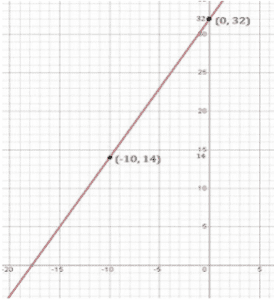Newbie

# In countries like USA and Canada, temperature is measured in Fahrenheit, whereas in countries like India, it is measured in Celsius. Here is a linear equation that converts Fahrenheit to Celsius:(i) Draw the graph of the linear equation above using Celsius for x-axis and Fahrenheit for y-axis.Q.8(1)

• 0

Today i am solving the chapter linear equations in two variables .Find the simplest solution of ncert class 9th solution of linear equations in two variables of exercise 4.3 question number 8(1). Sir please help me to solve this question in a easiest and best way. In countries like USA and Canada, temperature is measured in Fahrenheit, whereas in countries like India, it is measured in Celsius. Here is a linear equation that converts Fahrenheit to Celsius:(i) Draw the graph of the linear equation above using Celsius for x-axis and Fahrenheit for y-axis

Share

1. Solution:

(i) According to the question,

F = (9/5)C + 32

Solving the equation,

We get,

When C = 0, F = 32

When C = -10 , F = 14

The points to be plotted are (0, 32), (-10, 14)• 0